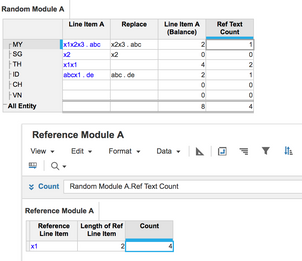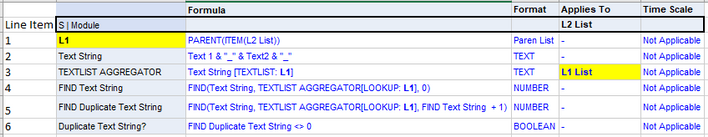# How to take the count of a text value that repeatsI have got a text field (column) say X, it has got values

x1

x2

x2

x3

wanted to creat a new line item to take the count with same value, for eg: x2 count will be 2 since it has got two entries.

Tagged:

•Hi anees,

I have created something simple, which you can use as a reference.

With the modules below, you can enter any text, and the formulas will look for the 'specific text' hidden with a long text.

You can also enter 'specific text' multiple times in the same cell.I have created 2 modules, i.e. Reference Module A, Random Module A.

Random Module A is the module where you have line items where you want to count the number of occurrences of 'specific text'.

Reference Module A is the module where you input the 'specific text' that you want to count, i.e. x1.

The formula of the different line items are:

Random Module A.Replace = SUBSTITUTE(Line Item A, Reference Module A.Reference Line Item, "")

Random Module A.'Line Item A (Balance)' = LENGTH(Line Item A) - LENGTH(Replace)

Random Module A.Ref Text Count = 'Line Item A (Balance)' / Reference Module A.Length of Ref Line Item

Reference Module A.Length of Ref Line Item = LENGTH(Reference Line Item)

Reference Module A.Count = Random Module A.Ref Text Count

A bit of description for how the different line items work together.

1. Random Module A.Replace looks for the 'specific text' and replace it with "", therefore nullifying the 'specific text'.

2. Random Module A.'Line Item A (Balance)' calculates the number of characters that have been replaced.

3. Random Module A.Ref Text Count calculates the number of times the 'specific text' appears.

Thanks,

LC

•Can someone please assist me with the above question?

•Hello

We do not have Count If function but if the said value is a valid list item belonging to a list we do have several workarounds.

•Hello

We do not have Count If function but if the said value is a valid list item belonging to a list we do have several workarounds.
•Thank you for the update harish 🙂

•Hello Anees,

one option would be to genereate a list, based on this line item.

So you will have a list that will say x1, x2, x3.

Then you can can create a second lineItem which has a FINDITEM('new list', text field) formula.

Then you need to create a third line items 'count' where you can put as a formula 1. So that it is always shows a 1.

Then you can create a count module. Which will have the 'new list' and you need to pick up the count lineitem and SUM on the second line item. This will give you the number of times the text has been inputted.

This value you then can retrieve in the original module.

Attached are some screenshots of the modules in table and blueprint.

•Hello Anees,

one option would be to genereate a list, based on this line item.

So you will have a list that will say x1, x2, x3.

Then you can can create a second lineItem which has a FINDITEM('new list', text field) formula.

Then you need to create a third line items 'count' where you can put as a formula 1. So that it is always shows a 1.

Then you can create a count module. Which will have the 'new list' and you need to pick up the count lineitem and SUM on the second line item. This will give you the number of times the text has been inputted.

This value you then can retrieve in the original module.

Attached are some screenshots of the modules in table and blueprint.

•The workarounds are good, but in some cases the additional line items/modules will increase the size more than desired. In the case of large data HUBS where these "counts" are needed for data validation purposes, a function similar to excel "count" would be very useful and help reduce model size and complexity.

•Hi, Please have the values in column as List i.e. create a list for the values in column (let's say list x)

Then create a line item(count) in module with Number format and value 1 .

count =1

Then create a line item with number format and use below formula:-

Count[SUM: x, LOOKUP: x]

This will capture the count. Hope this helps.

•The option above works but like you said there are many line items that factor into the formula.

The method below is for finding duplicate text fields using the aggregate x[TEXTLIST]y

Conditions that apply, the list is part of a hierarchy and a parent will be used to aggregate the text fields using: (Text.Line Item[TEXTLIST: Parent.Line Item[Lookup: Systems List Parent Line Item])1) The S | Systems modules line item to satisfy the L2 - L1 relationship for a Parent Lookup function.

2) Text String, or the series of text fields you wish to find duplicates of, in this case, there are two text fields separated by a delimiter "_" to separate the text string combinations.

3) This line item is Applied to the Parent of the L2 List applied to the Module. This formula uses the  x[TEXTLIST]y aggregate function to aggregate all of the L2 List text fields, input in the Text String Line Item (applied to L2) to its parent L1, separating the Text Strings with commas ( Text 1a_Text 2a , Text 1a_Text 2b, Text 1a, Text 2a

4) Line Item uses the FIND function to find the first instance of the Text String Aggregated to the L1 Parent, by looking up the TEXTLIST AGGREGATOR using the L1 systems line item, starting at point 0 of the text field.

5) This line item is used to find the SECOND instance of a text field or a single duplicate, by making the starting point of the FIND function, the number in Line item 4, + 1 . This means that if the first instance of the string is found in line item 4 that number position + 1 will be the starting point of the second formula, and if the formula is larger than 0, then there is a duplicate.

6) This is the BOOLEAN logic that indicates if a SINGLE DUPLICATE, or "Second Instance" of a text string is found.

This is not ideal for the "COUNT" of strings, as a line item will be needed to find each instance after the first duplicate, however, it is extremely powerful when creating items into a numbered lists and the names need to be unique and for unicity checks for unique code creation.

The TEXTLIST function is not the fastest performing function, as it concatenates text fields which are 8 bytes of memory, and is limited to 10,000 items in a list. I would suggest only using this for hierarchies with less than 1,000 items for performance purposes.

I hope this helps!

Tyler